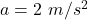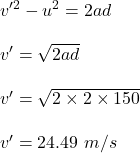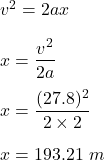Question

You are designing an airport for small planes. One kind of airplane that might use this airfield must reach a speed before takeoff of at least 27.8 m/s (100 km/h), and can accelerate at 2.00 m/s^2. a) If the runway is 150 meter long, can this airplane reach the required speed for takeoff? if not, what minimum length must the runway have?

1.Explanation:

Given that,

Initial speed of the airfield, u = 0

Final speed, v = 27.8 m/s

Acceleration of the airfield,Length of the runway, d = 150 m

Let v’ is the speed of the airplane to reach the required speed for takeoff. Finding v’ using third equation of motion as :This speed is not enough as the airfield must reach a speed before takeoff of at least 27.8 m/s. Now, the required length of the runways is :So, the minimum length of the runways is 193.21 meters.